# MCQ in DC Circuits Part 10 | REE Board Exam

(Last Updated On: November 13, 2020)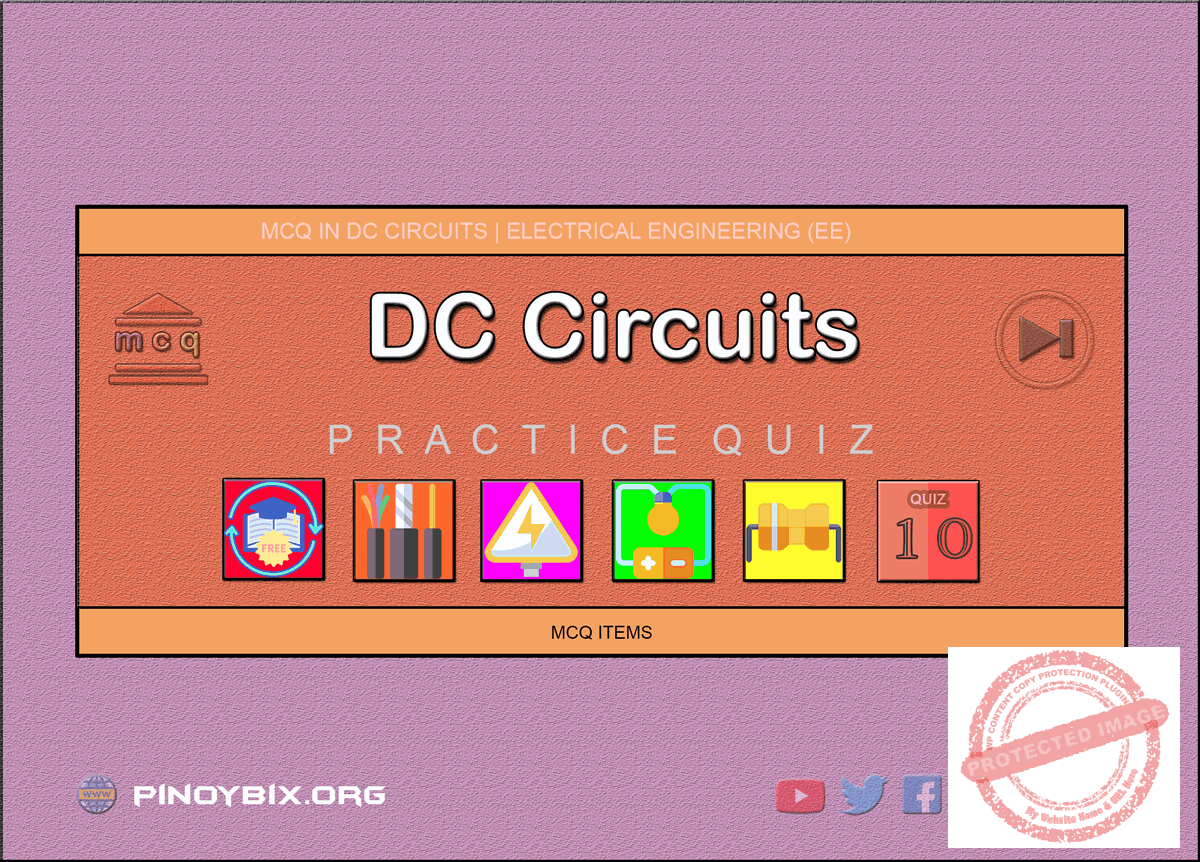This is the Multiple Choice Questions Part 10 of the Series in DC Circuits as one of the Electrical Engineering topic. In Preparation for the REE Board Exam make sure to expose yourself and familiarize in each and every questions compiled here taken from various sources including but not limited to past Board Exam Questions in Electrical Engineering field, Electrical Engineering Books, Journals and other Electrical Engineering References.

#### Continue Practice Exam Test Questions Part 10 of the Series

NETWORK THEOREMS

Choose the letter of the best answer in each questions.

451.  The value of Rth in Fig. 3.4(b) is ____.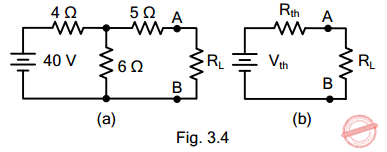A.  15 Ω

B.  3.5 Ω

C.  6.4 Ω

D.  7.4 Ω

Solution:

452.  The  open-circuited  voltage  at  terminals  AB  in  Fig. 3.4(a) isA.  12 V

B.  20 V

C.  24 V

D.  40 V

Solution:

453.  For transfer of maximum power in the circuit shown in Fig. 3.4(a), the value of RL should be ____.A.  3.5 Ω

B.  6.4 Ω

C.  7.4 Ω

D.  15 Ω

Solution:

454.  Fig. 3.5(b) shows Norton’s equivalent circuit of Fig. 3.5(a). The value of RN is ____.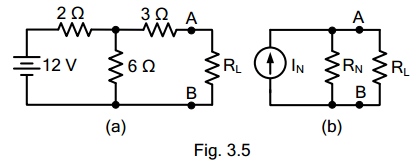A.  5 Ω

B.  4.5 Ω

C.  10.5 Ω

D.  none of these

Solution:

455.  The value of IN in Fig. 3.5(b) is ____.A.  3 A

B.  1 A

C.  2 A

D.  none of these

Solution:

456.  Thevenin’s  theorem  is  ____  form  on an equivalent circuit.

A.  voltage

B.  current

C.  both voltage and current

D.  none of these

Solution:

457.  Norton’s theorem is ____ Thevenin’s theorem.

A   the same as.

B.  converse of

C.  equal to

D.  none of these

Solution:

458.  In  the  analysis  of  a  vacuum  tube  circuit,  we generally use ____.

A.  superposition

B.  Norton’s

C.  Thevenin’s

D.  reciprocity

Solution:

459.  Norton’s  theorem  is  ____  form  of  an  equivalent circuit

A.  voltage

B.  current

C.  both voltage and current

D.  none of these

Solution:

460.  In  the  analysis  of  a  transistor  circuit,  we  generally use ____.

A.  Norton’s

B.  Thevenin’s

C.  reciprocity

D.  superposition

Solution:

461.  Fig.  3.6(a)  shows  Norton’s  equivalent  circuit  of  a network  whereas  Fig.  3.6(b)  shows  its  Thevenin’s equivalent circuit. The value of Vth is ____.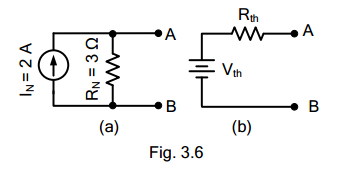A.  1.5 V

B.  0.866 V

C.  3 V

D.  6 V

Solution:

462.  The value of Rth in Fig. 3.6(b) is ____.A.  3 Ω

B.  2 Ω

C.  1.5 Ω

D.  6 Ω

Solution:

463.  If in Fig. 3.6(a), the value of IN  is 3 A, then value of Vth in Fig. 3.6(b) will be ____.A.  1 V

B.  9 V

C.  5 V

D.  none of these

Solution:

464.  For maximum power transfer, the relation between load resistance R and internal resistance Ri  of the voltage source is ____.

A.  RL = 2Ri

B.  RL = 0.5R

C.  RL = 1.5Ri

D.  RL = Ri

Solution:

465.  Under  the  conditions  of  maximum  power  transfer, the efficiency is ____.

A.  75%

B.  100%

C.  50%

D.  25%

Solution:

466.  The open-circuited voltage at terminals of load RL is 30  V  Under  the  conditions  of  maximum  power transfer, the load voltage would be ____.

A.  30 V

B.  10 V

C.  5 V

D.  15 V

Solution:

467.  The  maximum  power  transfer  theorem  is  used  in ____.

A.  electronic circuits

B.  power system

C.  home lighting circuits

D.  none of these

Solution:

468.  Under the conditions of maximum power transfer, a voltage source is delivering a power of 30 W to the load. The power generated by the source is ____.

A.  45 W

B.  30 W

C.  60 W

D.  90 W

Solution:

469.  For  the  circuit  shown  in  Fig.  3.7,  the  power transferred  will  be  maximum  when  RL  is  equal  to _____.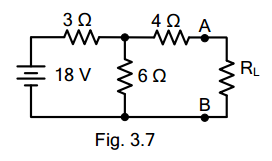A.  4.5 Ω

B.  6 Ω

C.  3 Ω

D.  none of these

Solution:

470.  The  open-circuited  voltage  at  terminals  AB  in  Fig. 3.7 is ____.A.  12 V

B.  6 V

C.  15 V

D.  9.5 V

Solution:

471.  If  in  Fig.  3.7,  the  value  of  RL  =  6  Ω,  then  current through RL is ____.A.  2 A

B.  1.5 A

C.  1.75 A

D.  1 A

Solution:

472.  Under  the  conditions  of  maximum  power  transfer, the voltage across RL in Fig. 3.7 is ____.A.  6 V

B.  4 V

C.  9 V

D.  12 V

Solution:

473.  The output resistance of a voltage source is 4 Ω. Its internal resistance will be ____.

A.  4 Ω

B.  2 Ω

C.  1 Ω

D.  infinite

Solution:

474.  Delta/star  of  star/delta  transformation  technique  is applied to _____.

A.  one terminal

B.  two terminal

C.  three terminal

D.  none of these

Solution:

475.  Kirchhoff’s current law is applicable to only

A.   closed loops in a network

B.  electronic circuits

C.  conjunctions in a network

D.  electric circuits

Solution:

476.  Kirchhoff’s voltage law is concerned with

A.  IR drops

B.  battery e.m.f.s.

C.  junction voltages

D.  both A and B

Solution:

477.  According to KVL, the algebraic sum of all IR drops and  e.m.f.s  in  any  closed  loop  of  a  network  is always

A.  zero

B.  positive

C.  negative

D.  determined by the battery e.m.f.s

Solution:

478.  The  algebraic  sign  of  an  IR  drop  is  primarily dependent upon the

A.  amount of current flowing through it

B.  value of R

C.  direction of current flow

D.  battery connection

Solution:

479.  Maxwell’s  loop current  method  of solving  electrical networks

A.  uses branch currents

B.  utilizes Kirchhoff’s voltage law

C.  is confined to single-loop circuits

D.  is a network reduction method

Solution:

480.  Point  out  the  WRONG  statement.  In  the  node-voltage  technique  of  solving  networks,  choice  of  a reference node does not

A.  affect the operation of the circuit

B.  change the voltage across any element

C.  alter the p.d. between any pair of nodes

D.  affect the voltages of various nodes

Solution:

481.  The  nodal  analysis  is  primarily  based  on  the application of

A.  KVL

B.  KCL

C.  Ohm’s Law

D.  both B and C

Solution:

482.  Superposition  theorem  is  can  be  applied  only  to circuits having ____ elements.

A.  non-linear

B.  passive

C.  linear bilateral

D.  resistive

Solution:

483.  The Superposition theorem is essentially based on the concept of

A.  duality

B.  linearity

C.  reciprocity

D.  non-linearity

Solution:

484.  While Thevenizing a circuit between two terminals, Vth equals

A.  short-circuit terminal voltage

B.  open circuit terminal voltage

C.  EMF of the battery nearest to the terminal

D.  net voltage available in the circuit

Solution:

485.  Thevenin resistance Rth is found

A.  between any two “open” terminals

B.  by short-circuiting the given two terminals

C.   by  removing  voltage  sources  along  with  their internal resistance

D.  between same open terminals as for Vth

Solution:

486.  While  calculating  Rth,  constant-current  sources  in the circuit are

A.  replaced by “opens”

B.  replaced by “shorts”

C.  treated in parallel with other voltage sources

D.  converted into equivalent voltage sources

Solution:

487.  Thevenin resistance of the circuit of  Fig. 2.1 across its terminals A and B is ____ ohm.A.  6

B.  3

C.  9

D.  2

Solution:

488.  The  load  resistance  needed  to  extract  maximum power from the circuit of Fig. 2.2 is ____ ohm.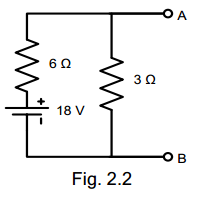A.  2

B.  9

C.  6

D.  18

Solution:

489.  The Norton equivalent circuit for the network of Fig. 2.2  between  A  and  B  is  ____  current  source  with parallel resistance of ____.A.  2 A, 6 Ω

B.  3 A, 2 Ω

C.  2 A, 3 Ω

D.  3 A, 9 Ω

Solution:

490.  The Norton equivalent of a circuit consists of a 2 A current  source  in  parallel  with  a  4 Ω  resistor. Thevenin  equivalent  of  this  circuit  is  a  ____  volt source in series with a 4 Ω resistor.

A.  2

B.  0.5

C.  6

D.  8

Solution:

491.  If  two  identical  3  A,  4  Ω Norton  equivalent  circuits are  connected  in  parallel  with  like  polarity  to  like, the combined Norton equivalent circuit is

A.  6 A, 4 Ω

B.  6 A, 2 Ω

C.  3 A, 2 Ω

D.  6 A, 8 Ω

Solution:

492.  Two  6  V,  2  Ω batteries  are  connected  in  series aiding.  This  combination  can  be  replaced  by  a single  equivalent  current  generator  of  ____  with  a parallel resistance of ____ ohm.

A.  3 A, 4 Ω

B.  3 A, 2 Ω

C.  3 A, 1 Ω

D.  5 A, 2 Ω

Solution:

493.  Two  identical  3  A,  1  Ω batteries  are  connected  in parallel  with  like  polarity  with  like  polarity  to  like. The Norton equivalent circuit of the combination is

A.  3 A, 0.5 Ω

B.  6 A, 1 Ω

C.  3 A, 1 Ω

D.  6 A, 0.5 Ω

Solution:

494.  Thevenin equivalent circuit of the network shown in Fig.  2.3  is  required.  The  value  of  the  open-circuit voltage  across  terminals  a  and  b  of  this  circuit  is ____ volt.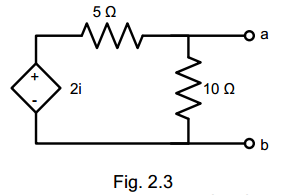A.  zero

B.  2i/10

C.  2i/5

D. 2i/15

Solution:

495.  For  a  linear  network  containing  generators  and impedance,  the  ratio  of  the  voltage  to  the  current produced  in  other  loop  is  the  same  as  the  ratio  of voltage and  current obtained when the positions of the voltage source and the ammeter measuring the current  are  interchanged.  This  network  theorem  is known as ____ theorem.

A.  Millman’s

B.  Norton’s

C.  Tellegen’s

D.  Reciprocity

Solution:

496.  A  12  volt  source  with  an  internal  resistance  of  1.2 ohms  is  connected  across  a  wire-wound  resistor. Maximum  power  will  be  dissipated  in  the  resistor when its resistance is equal to

A.  zero

B.  1.2 ohm

C.  12 ohms

D.  infinity

Solution:

497.  Three 3.33 Ω  resistors are connected in wye. What is the value of the equivalent resistors connected in delta?

A.  3.33 Ω

B.  10 Ω

C.  6.67 Ω

D.  20 Ω

Solution:

498.  Find the equivalent resistance between terminals a &  b  of  the  circuit  shown.   Each  resistance  has  a value of 1 ohm.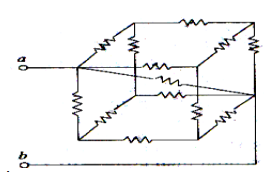A.  5/6 ohms

B.  5/11 ohms

C.  5/14 ohms

D.  5/21 ohms

Solution:

499.  What do you call the head to tail connection of two or more op-amp circuits wherein the output of one op-amp is the input of another op-amp?

A.  Parallel Op-Amps

B.  Follow-Thru Connection

D.  Series Op-Amps

Solution:

500.  Find the power dissipation in the 6 ohms resistor in the next figure.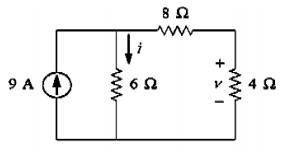A.  54 W

B.  216 W

C.  121.5 W

D.  150 W

Solution:

#### Questions and Answers in DC Circuits

Following is the list of practice exam test questions in this brand new series:

MCQ in DC Circuits
PART 1: MCQ from Number 1 – 50                Answer key: included
PART 2: MCQ from Number 51 – 100            Answer key: included
PART 3: MCQ from Number 101 – 150           Answer key: included
PART 4: MCQ from Number 151 – 200           Answer key: included
PART 5: MCQ from Number 201 – 250           Answer key: included
PART 6: MCQ from Number 251 – 300           Answer key: included
PART 7: MCQ from Number 301 – 350           Answer key: included
PART 8: MCQ from Number 351 – 400            Answer key: included
PART 9: MCQ from Number 401 – 450            Answer key: included
PART 10: MCQ from Number 451 – 500          Answer key: included

P inoyBIX educates thousands of reviewers and students a day in preparation for their board examinations. Also provides professionals with materials for their lectures and practice exams. Help me go forward with the same spirit.

“Will you subscribe today via YOUTUBE?”

Subscribe

PinoyBIX Engineering. © 2014-2020 All Rights Reserved | How to Donate? |